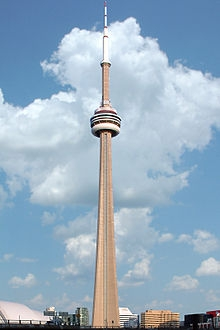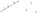# An observer

An observer standing west of the tower sees its top at an altitude angle of 45 degrees. After moving 50 meters to the south, he sees its top at an altitude angle of 30 degrees. How tall is the tower?

Correct result:

y =  35.355 m

#### Solution:

$A=45 \ ^\circ \ \\ B=30 \ ^\circ \ \\ x_{3}=50 \ \text{m} \ \\ \ \\ \tan A=y : x_{1} \ \\ \tan B=y : x_{2} \ \\ \ \\ x_{2}^2=x_{3}^2+x_{1}^2 \ \\ \ \\ y=x_{1} \cdot \ \tan A=x_{2} \cdot \ \tan B=35.355 \ \text{m} \ \\ \ \\ x_{1} \cdot \ \tan A=x_{2} \cdot \ \tan B \ \\ x_{1}^2 \cdot \ \tan^2 \ A=x_{2}^2 \cdot \ \tan^2 \ B \ \\ \ \\ x_{1}^2 \cdot \ \tan^2 \ A=(x_{3}^2+x_{1}^2) \cdot \ \tan^2 \ B \ \\ \ \\ t_{1}=(\tan A ^\circ )^2=(\tan 45^\circ \ )^2=1^2=1 \ \\ t_{2}=(\tan B ^\circ )^2=(\tan 30^\circ \ )^2=0.57735^2=0.33333 \ \\ \ \\ x_{1}^2 \cdot \ t_{1}=x_{3}^2 \cdot \ t_{2} +x_{1}^2 \cdot \ t_{2} \ \\ x_{1}^2 \cdot \ (t_{1}-t_{2})=x_{3}^2 \cdot \ t_{2} \ \\ \ \\ x_{1}=x_{3} \cdot \ \sqrt{ \dfrac{ t_{2} }{ t_{1}-t_{2} } }=50 \cdot \ \sqrt{ \dfrac{ 0.3333 }{ 1-0.3333 } } \doteq 25 \ \sqrt{ 2 } \ \text{m} \doteq 35.3553 \ \text{m} \ \\ \ \\ y=x_{1} \cdot \ \sqrt{ t_{1} }=35.3553 \cdot \ \sqrt{ 1 } \doteq 25 \ \sqrt{ 2 } \doteq 35.3553 \ \\ \ \\ \text{ Correctness test: } \ \\ x_{2}=y / \sqrt{ t_{2} }=35.3553 / \sqrt{ 0.3333 } \doteq 25 \ \sqrt{ 6 } \ \text{m} \doteq 61.2372 \ \text{m} \ \\ \ \\ x_{22}=\sqrt{ x_{3}^2+x_{1}^2 }=\sqrt{ 50^2+35.3553^2 } \doteq 25 \ \sqrt{ 6 } \ \text{m} \doteq 61.2372 \ \text{m}$Our examples were largely sent or created by pupils and students themselves. Therefore, we would be pleased if you could send us any errors you found, spelling mistakes, or rephasing the example. Thank you!

Leave us a comment of this math problem and its solution (i.e. if it is still somewhat unclear...):Be the first to comment!Tips to related online calculators
Pythagorean theorem is the base for the right triangle calculator.

#### You need to know the following knowledge to solve this word math problem:

We encourage you to watch this tutorial video on this math problem:

## Next similar math problems:

• Find the 10Find the value of t if 2tx+5y-6=0 and 5x-4y+8=0 are perpendicular, parallel, what angle does each of the lines make with the x-axis, find the angle between the lines?
• Three points 2The three points A(3, 8), B(6, 2) and C(10, 2). The point D is such that the line DA is perpendicular to AB and DC is parallel to AB. Calculate the coordinates of D.
• Decide 2Decide whether points A[-2, -5], B[4, 3] and C[16, -1] lie on the same line
• Angle of the body diagonalsUsing vector dot product calculate the angle of the body diagonals of the cube.
• Two peopleTwo straight lines cross at right angles. Two people start simultaneously at the point of intersection. John walking at the rate of 4 kph in one road, Jenelyn walking at the rate of 8 kph on the other road. How long will it take for them to be 20√5 km apa
• Right angled triangle 2LMN is a right angled triangle with vertices at L(1,3), M(3,5) and N(6,n). Given angle LMN is 90° find n
• CuboidsTwo separate cuboids with different orientation in space. Determine the angle between them, knowing the direction cosine matrix for each separate cuboid. u1=(0.62955056, 0.094432584, 0.77119944) u2=(0.14484653, 0.9208101, 0.36211633)
• CoordinatesDetermine the coordinates of the vertices and the content of the parallelogram, the two sides of which lie on the lines 8x + 3y + 1 = 0, 2x + y-1 = 0 and the diagonal on the line 3x + 2y + 3 = 0
• Vector perpendicularFind the vector a = (2, y, z) so that a⊥ b and a ⊥ c where b = (-1, 4, 2) and c = (3, -3, -1)
• Vector equationLet’s v = (1, 2, 1), u = (0, -1, 3) and w = (1, 0, 7) . Solve the vector equation c1 v + c2 u + c3 w = 0 for variables c1 c2, c3 and decide weather v, u and w are linear dependent or independent
• Coordinates of a centroindLet’s A = [3, 2, 0], B = [1, -2, 4] and C = [1, 1, 1] be 3 points in space. Calculate the coordinates of the centroid of △ABC (the intersection of the medians).
• Parametric formCalculate the distance of point A [2,1] from the line p: X = -1 + 3 t Y = 5-4 t Line p has a parametric form of the line equation. ..
• Set of coordinatesConsider the following ordered pairs that represent a relation. {(–4, –7), (0, 6), (5, –3), (5, 2)} What can be concluded of the domain and range for this relation?
• Coordinates of square verticesThe ABCD square has the center S [−3, −2] and the vertex A [1, −3]. Find the coordinates of the other vertices of the square.
• Find the 5Find the equation with center at (1,20) which touches the line 8x+5y-19=0
• Right triangle from axesA line segment has its ends on the coordinate axes and forms with them a triangle of area equal to 36 square units. The segment passes through the point ( 5,2). What is the slope of the line segment?
• Points collinearShow that the point A(-1,3), B(3,2), C(11,0) are col-linear.